## A tank has the shape of an inverted circular cone with height 8 m andbase radius 2 m. It is filled with water to the top. Find the integralf

Question

A tank has the shape of an inverted circular cone with height 8 m andbase radius 2 m. It is filled with water to the top. Find the integralfor the work required to empty the tank by pumping all of the waterto the top of the tank. DoNOTcompute the integral. (The densityis 1000 kg/m3.)

in progress 0
2 months 2021-07-22T15:56:56+00:00 1 Answers 3 views 0

Step-by-step explanation:

I’ll try to make this make as much sense as possible. If we have a cone with a liquid in it, this liquid takes up volume. Therefore, our main equation, at least at first, is to find the volume. This is because if we pump the liquid out of the tank, the thing that changes is the amount of liquid in the tank which is the tank’s volume. The formula for the volume of a circular cone is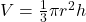and here’s what we know:

r = 2 and h = 8. The formula for volume has too many unknowns in it, so let’s get the radius in terms of the height and sub that in so we only have one variable. The reason I’m getting rid of the radius is because in the problem we are being asked how much work is done by pumping the liquid to the top of the tank, which is a height thing. Solve for r in terms of h using proportions: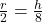and solve for r: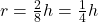so we will plug that in and rewrite the equation: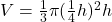and simplify it til it’s a simple as it can get.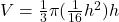and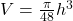and since the volume is what is changing as we pump liquid out, we find the derivative of this equation.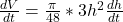and of course this simplifies as well: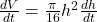Work is equal to the amount of force it takes to move something times the distance it moves. In order to find the force it takes to move this liquid, we need to multiply the amount (volume) of liquid times the weight of it, given as 1000 kg/m³:

F =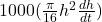and the distance it moves is 8 – h since the liquid has to move the whole height of the tank in order to move to the top of the 8-foot tank. That makes the whole integral become: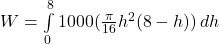and we’ll just simplify it down all the way: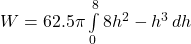and you’re done (except for solving it, which is actually the EASY part!!)